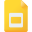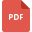Estimate Sums and DifferencesAddition and Multiplication Properties for Real NumbersAdding and Subtracting Fractions Unlike DenominatorsEstimating Sum and Difference of Decimal NumbersSolving Equations containing Addition and Subtraction WorksheetBasic Math, Grade 1, Australia, Fundamental Math or Basic MathAdding and Subtracting Fractions with Sample QuestionsDirected Numbers: Addition and Subtraction WorksheetsAddition, Subtraction, Multiplication, Division of Fractions
Fraction, Grade 5, United States, MathematicsAddition and Multiplication Properties for Real NumbersDecimals and Division, Grade 6, United States, Decimals and MultiplicationProbability, Grade 6, United States, MathematicsAdding Decimals upto 3 Decimal Places